Select Page

# Three Dimensional Geometry MCQ CBSE Maths 12 Science Solutions in English

Three Dimensional Geometry MCQ CBSE Maths 12 Science Solutions in English to enable students to get Solutions in a narrative video format for the specific question.

Expert Teacher provides Three Dimensional Geometry MCQ CBSE Maths 12 Science Solutions through Video Solutions in English language. This video solution will be useful for students to understand how to write an answer in exam in order to score more marks. This teacher uses a narrative style for a question from Three Dimensional Geometry not only to explain the proper method of answering question, but deriving right answer too.

Please find the question below and view the Solution in a narrative video format.

Question:

Solution Video in English:

## Similar Questions from CBSE, 12th Science, Maths, Three Dimensional Geometry

Question 1 : If a line has direction ratios 2, -1, -2, then what are its direction cosines? (View Answer Video)

Question 2 : Find the co-ordinates of the point where the line through the points A (3, 4, 1) and B (5, 1, 6) crosses the XY-plane. (View Answer Video)

Question 3 : Find the vector equation of the plane with intercepts 3, -4 and 2 on x, y and z-axis respectively. (View Answer Video)

Question 4 : If a line makes angle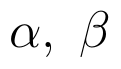and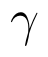with the coordinate axis, then find the value of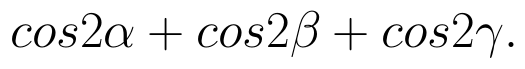(View Answer Video)

### Continuity and Differentiability

Question 1 : Using the fact that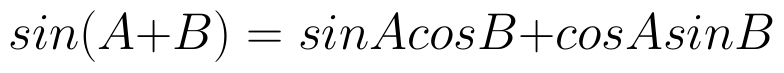and the differentiation, obtain the sum formula for cosines. (View Answer Video)

Question 2 : Differentiate the function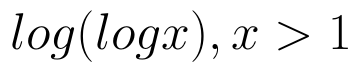with respect to x. (View Answer Video)

Question 3 : Findfor the function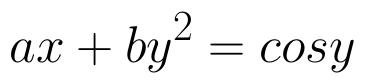. (View Answer Video)

Question 4 : Differentiate the function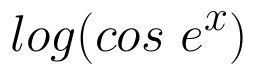with respect to x. (View Answer Video)

Question 5 : Differentiate the function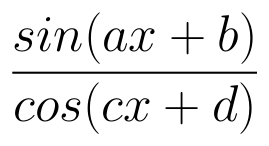with respect to x. (View Answer Video)

### Differential Equations

Question 1 : Write the differential equation obtained by eliminating the arbitrary constant C in the equation representing the family of curves xy = C cos x. (View Answer Video)

Question 2 : Find the particular solution of the differential equation :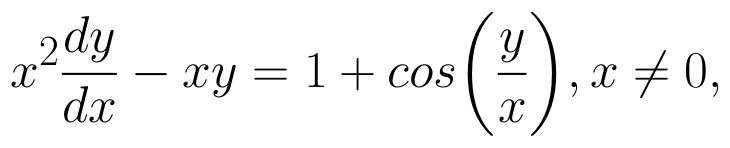when x = 1,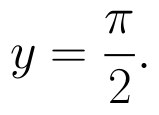(View Answer Video)

Question 3 : Write the differential equation representing the family of curves y = mx, where m is an arbitrary constant. (View Answer Video)

Question 4 : Form the differential equation of equation y = a cos 2x + b sin 2x, where a and b are constant. (View Answer Video)

Question 5 : Write the degree of the differential equation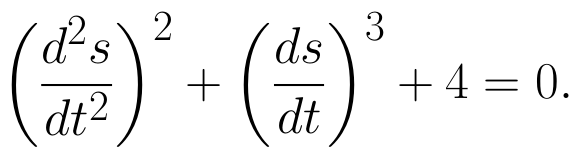(View Answer Video)

### Application of Integrals

Question 1 : Find the area of the region bounded by the curve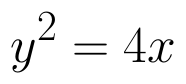and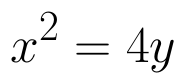. (View Answer Video)

Question 2 : Find the area of the given curves and given lines: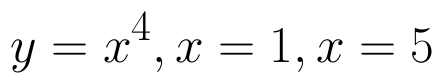and x-axis (View Answer Video)

Question 3 : Find the area of the region bounded by the parabola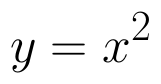and y = | x |. (View Answer Video)

Question 4 : Find the area of the smaller region bounded by the ellipse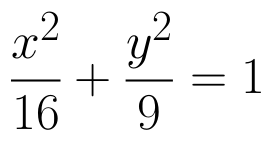and the straight line 8x + 3y = 12. (View Answer Video)

Question 5 : Find the area of smaller region bounded by the ellipse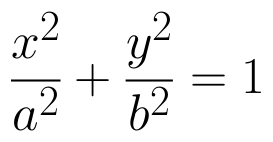and the line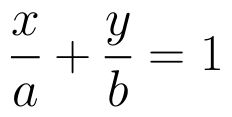. (View Answer Video)